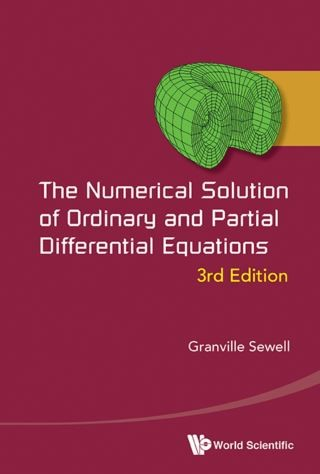## The Numerical Solution of Ordinary and Partial Differential Equations• Description
• Author
• Info
• Reviews

### Description

This book presents methods for the computational solution of differential equations, both ordinary and partial, time-dependent and steady-state. Finite difference methods are introduced and analyzed in the first four chapters, and finite element methods are studied in chapter five. A very general-purpose and widely-used finite element program, PDE2D, which implements many of the methods studied in the earlier chapters, is presented and documented in Appendix A.

The book contains the relevant theory and error analysis for most of the methods studied, but also emphasizes the practical aspects involved in implementing the methods. Students using this book will actually see and write programs (FORTRAN or MATLAB) for solving ordinary and partial differential equations, using both finite differences and finite elements. In addition, they will be able to solve very difficult partial differential equations using the software PDE2D, presented in Appendix A. PDE2D solves very general steady-state, time-dependent and eigenvalue PDE systems, in 1D intervals, general 2D regions, and a wide range of simple 3D regions.

Contents:
• Direct Solution of Linear Systems
• Initial Value Ordinary Differential Equations
• The Initial Value Diffusion Problem
• The Initial Value Transport and Wave Problems
• Boundary Value Problems
• The Finite Element Methods
• Appendix A — Solving PDEs with PDE2D
• Appendix B — The Fourier Stability Method
• Appendix C — MATLAB Programs
• Appendix D — Answers to Selected Exercises